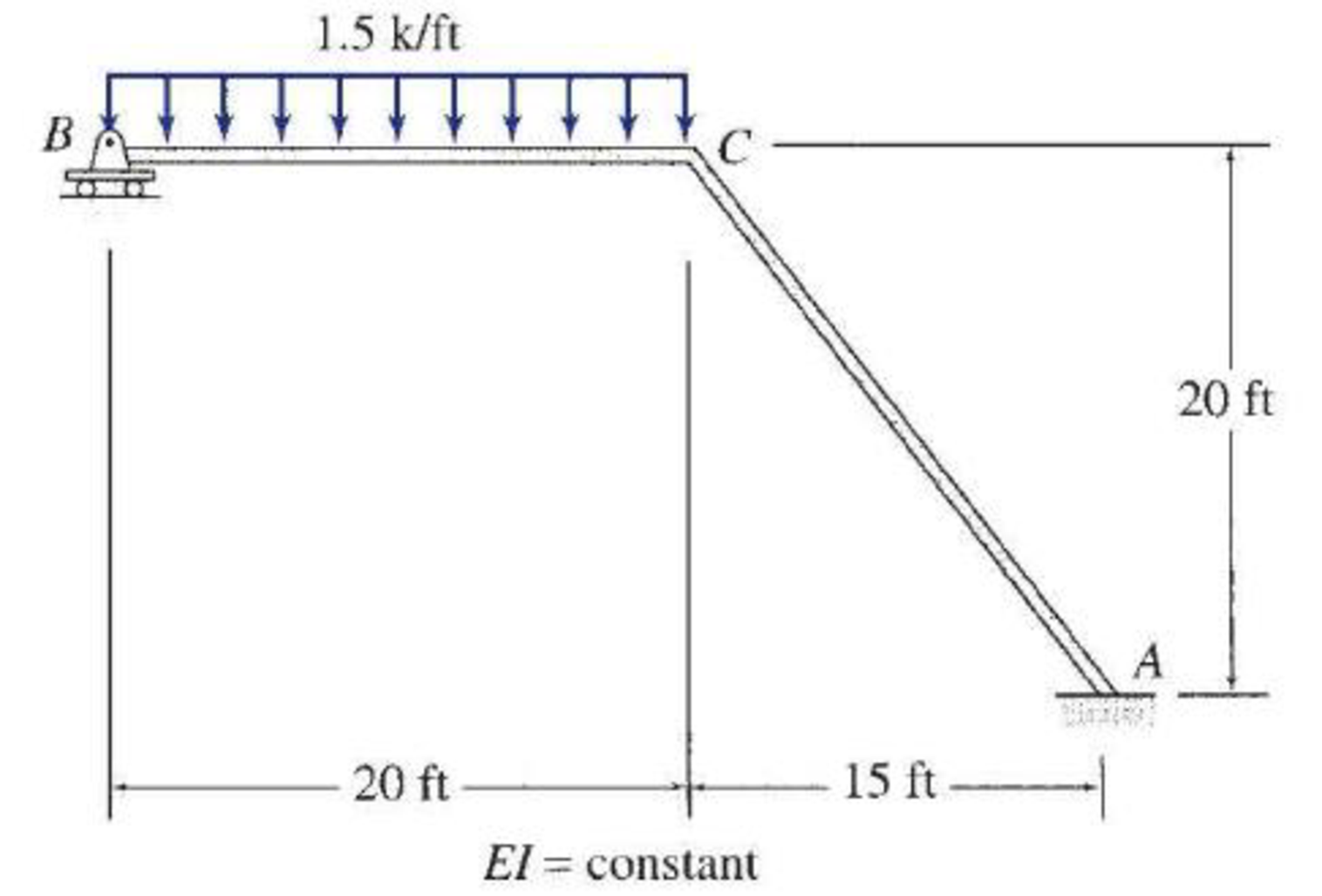# Determine the member end moments and reactions for the frames shown in Figs. P16.24–P16.31 by using the moment-distribution method. FIG. P16.28

#### Solutions

Chapter
Section
Chapter 16, Problem 28P
Textbook Problem
1 views

## Determine the member end moments and reactions for the frames shown in Figs. P16.24–P16.31 by using the moment-distribution method.FIG. P16.28

To determine

Find the member end moments and reactions for the frames.

### Explanation of Solution

Fixed end moment:

Formula to calculate the relative stiffness for fixed support IL and for roller support (34)(IL).

Formula to calculate the fixed moment for point load with equal length are PL8.

Formula to calculate the fixed moment for point load with unequal length are Pab2L2 and Pa2bL2.

Formula to calculate the fixed moment for UDL is WL212.

Formula to calculate the fixed moment for UVL are WL230 and WL220.

Formula to calculate the fixed moment for deflection is 6EIΔL2

Calculation:

Show the free body diagram of the entire frame as in Figure 1.

Refer Figure 1,

Calculate the length of the member AC:

LAC=202+152=400+225=25ft

Calculate the relative stiffness KCB for member CB of the frame as below:

KCB=(34)I20=3I80

Calculate the relative stiffness KCA for member CA of the frame as below:

KCA=I25

Calculate the distribution factor DFCB for member CB of the frame.

DFCB=KCBKCB+KCA

Substitute 3I80 for KCB and I25 for KCA.

DFCB=3I803I80+I25=0.484

Calculate the distribution factor DFCA for member CA of the frame.

DFCA=KCAKCB+KCA

Substitute 3I80 for KCB and I25 for KCA.

DFCA=I253I80+I25=0.516

Check for sum of distribution factor as below:

DFCB+DFCA=1

Substitute 0.484 for DFBA and 0.516 for DFBC.

0.484+0.516=1

Hence, OK.

Calculate the fixed end moment for BC.

FEMBC=1.5×(20)212=50kft

Calculate the fixed end moment for CB.

FEMCB=1.5×(20)212=50kft

Calculate the fixed end moment for CA and AC.

FEMCA=FEMAC=0

Show the calculation of MO moments using moment distribution method for side-sway prevented as in Table 1.

Show the free body diagram of the member BC and CA with unknown load R as in Figure 2.

Consider member BC:

Calculate the vertical reaction at the joint B by taking moment about point C.

+MB=0Cy1(20)38.7(1.5×20×202)=0Cy1(20)=338.7Cy1=338.720Cy1=16.94k

Calculate the vertical reaction at joint B by resolving the horizontal equilibrium.

+Fy=0Cy1+By1(1.5×20)=016.94+By130=0By1=13.06k

Consider member CA

Calculate vertical reaction at joint A:

Ay1=Cy1=16.94k

Calculate horizontal reaction at joint A by taking moment about point B.

+MB=0Ax1(20)+(16.94×15)+38.7+19.4=0Ax1(20)=312.2Ax1=312.220Ax1=15.61k

Calculate the horizontal reaction at joint C by resolving the horizontal equilibrium.

+Fx=0Ax1+Bx1=015.61+Bx1=0Cx1=15.61k

Calculate the horizontal reaction at joint B:

Bx1=Cx1=15.61k

Calculate the reaction R:

R=Bx1=15.61k

Show the arbitrary translation as in Figure 3.

Calculate the relative translation ΔBC between the ends of member BC:

ΔBC=(34)Δ=0.75Δ

Calculate the relative translation ΔAC between the ends of member AC:

ΔAC=(54)Δ=1.25Δ

Calculate the fixed end moment for BC.

FEMBC=6EI(ΔBC)(20)2

Substitute 0.75Δ for ΔAB.

FEMBC=6EI(0.75Δ)(20)2 (1).

Calculate the fixed end moment for CB.

FEMCB=6EI(0.75Δ)(20)2

Calculate the fixed end moment for CA.

FEMCA=6EI(ΔAC)(25)2

Substitute 1.25Δ for ΔAC.

FEMCA=6EI(1.25Δ)(25)2        (2)

Calculate the fixed end moment for CB.

FEMCB=6EI(1.25Δ)(25)2

Assume the Fixed-end moment at BC, and CB as 100kft

FEMBC=FEMCB=100kft

Calculate the value of EIΔ using the equation (1)

### Still sussing out bartleby?

Check out a sample textbook solution.

See a sample solution

#### The Solution to Your Study Problems

Bartleby provides explanations to thousands of textbook problems written by our experts, many with advanced degrees!

Get Started

Find more solutions based on key concepts
What is the difference between engineering and engineering technology?

Engineering Fundamentals: An Introduction to Engineering (MindTap Course List)

What e the main principles of source document design?

Systems Analysis and Design (Shelly Cashman Series) (MindTap Course List)

What is OLE-DB used for, and how does it differ from ODBC?

Database Systems: Design, Implementation, & Management

Define the term, management information system (MIS). Differentiate among the three types of reports an MIS gen...

Enhanced Discovering Computers 2017 (Shelly Cashman Series) (MindTap Course List)

What does GDT stand for?

Precision Machining Technology (MindTap Course List)

What is the purpose of postheating the metal after welding?

Welding: Principles and Applications (MindTap Course List)

If your motherboard supports ECC DDR3 memory, can you substitute non-ECC DDR3 memory?

A+ Guide to Hardware (Standalone Book) (MindTap Course List)